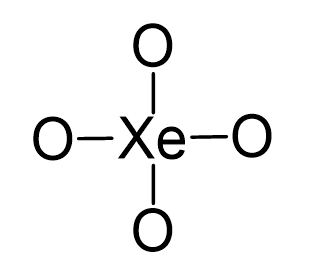## Examples

Xe is the central atom, so we can draw a preliminary skeletal structure:There are 4×6 + 8 = 32 electrons and this time, instead of putting three lone pairs on the oxygen, we are going to directly add double bonds to leave two lone pairs for each oxygen:All the electrons are used: 4 double bonds = 16, 8 lone pairs = 16.

The central atom has four atoms and no lone pair, therefore, both the electron and molecular geometries are tetrahedral.Steric number 4 corresponds to sp3-hybridization where the idealized bond angles are 109.5o.

Check Also